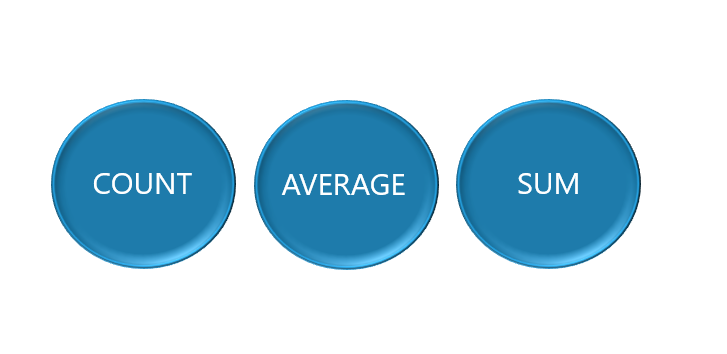# Using Aggregate FunctionsIn a previous post, we discussed the concepts of functions and using scalar functions. In this post, we will focus on aggregate functions that are used in SQL to take a series of values and return a singular result. For example, in the AdventureWorks database, there is a Sales.SalesOrderHeader table that has 31, 465 records, here is an example of the first nine records. (Note: we are only selecting four columns from the table, but there are twenty-five columns in this table.)

``````SELECT SalesOrderID, OrderDate, Freight, TotalDue

Next, we are going to aggregate the data for each of these columns. Each aggregate function accepts a single parameter, more specifically the series of values from a column that needs to be aggregated.

``````SELECT COUNT(SalesOrderID) as CountOrders,
MAX(OrderDate) as MaxOrderDate,
AVG(Freight) as AverageFreight,
SUM(TotalDue) as SumTotalDue